All submissions of the EM system will be redirected to Online Manuscript Submission System. Authors are requested to submit articles directly to Online Manuscript Submission System of respective journal.

# Short communication

, Volume: 7( 1) DOI: 10.37532/2320-6756.2019.7(1).172

## Insight on the Emergence of Classicality from Quantum-Ness

*Correspondence:
Ghosh AK Ph.D. (Physics), Bhagwant University, India, E-Mail: ghosh.971@gmail.com

Received: October 31, 2018; Accepted: December 28, 2018; Published: January 8, 2019

Citation: Ghosh AK. Insight on the Emergence of Classicality from Quantum-Ness. J Phys Astron. 2019;7(1):172.

### Abstract

Classical object has wave nature and wave-particle duality compounds confusion. On the contrary, quantum object is a coherent complex wave function which is probabilistic in nature. Classical and quantum interference differ by the presence of intermediate quantum interference terms which give rise to measurement problem. GRW theory, Pilot-wave formulation of quantum mechanics, Von Neumann, Zurek, Wheeler, Born’s principle, and also many physicists have worked on measurement problem. According to decoherence theory, quantum alternatives vanish due to the effect of entanglement with the environment. In this paper, I assume the effect of observer’s mind with respect to measuring device in order to explain classical behaviour from quantum-ness

### Keywords

Quantum-ness; Decoherence theory; Transition

### Introduction

Copenhagen interpretation said: there is common sense (classical) and there is quantum sense, but you can’t have both-at least, not at the same time.

Quantum processes are probabilistic and irreversible. They are different from classical measurements. Quantum theory explains the interactions between the object and the measurement apparatus. In classical physics, the observer registers the state of the real world without perturbing the observed system. But this cannot give a satisfactory answer to the basic question as regard to the constraints put on the results of physical measurements.

Quantum measurement has been discussed by J. von Neumann in his 1932 book and by decoherence phenomenon. The famous Solvay 1927 Congress and the 1983 review by Wheeler and Zurek thaught about measurement problem.

According to Born principle, the outcome of the measurement of an observable A on a state ψ is non-deterministic. Quantum mechanics give only probabilities and in particular the expectation value of the outcomes. This expectation value is given by the Born rule.These measurements can be repeated and performed independently.

The probability to get the outcome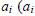being the eigenvalue of A andthe corresponding eigenvector) is the modulus squared of the probability amplitude i|ψ probability of outcome of A = ai in the state.

It also indicates that quantum measurements are an irreversible process. In ideal measurements, this can be repeated. This is
the projection postulate.

According to the concept of hidden variables and “element of reality” the quantum state of a quantum system could be
explained by some hidden variables in some space. This idea was rejected by most physicist after 1927 Solvay Congress and

According to the Von Neumann paradigm, an ideal measurement is an interaction between the quantum state (e.g. a q-bit )
and measurement apparatus. An ideal non-destructive measurement does not change the orthogonal states ofandof a
quantum system. This is also a projection postulate, and hence this plays a crucial but controversial role. This means that this
postulate has a conflict with Schrödinger’s equation.

Thus, the ambiguity of quantum measurement has been a controversial problem for a long time .

In the context of classical and quantum interference, the probabilistic nature of wave function gives rise to various quantum
effects [2-4].

The probability of observing the system making the transition from state Ψ to the new state ɸ is given by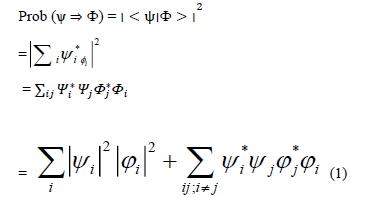Whereare the coefficients of the final state of the system * is the complex conjugate so that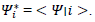Now, if the system transit classically fromvia an intermediate statethen we get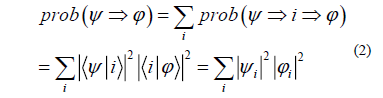Therefore, from equations (1) and (2) we can see that quantum equation differ from the classical equation by the extra terms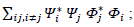these are the quantum alternative states [5-11].

According to decoherence theory, these alternatives vanish due to the effect of entanglement of the environment.

#### Conclusion

Let me present my imagination (where I have failed to make contact with standard quantum theory and the measurement axioms of quantum theory) is that the quantum alternative states are produced out of phase with each other in the plane of the observer’s mind with respect to the measuring device, and hence they are canceled out due to destructive interference among them and the single classical entity is left undisturbed. This might be a description as to how to get the single entity from quantum-ness.

### References

Welcome Message

###### Citations : 291

Journal of Physics & Astronomy received 291 citations as per Google Scholar report

#### Indexed In

• Open J Gate
• China National Knowledge Infrastructure (CNKI)
• Cosmos IF
• Directory of Research Journal Indexing (DRJI)
• MIAR
• Secret Search Engine Labs
• Euro Pub
• clarivate-web-of-science-logo-vector
• ICMJE

View More

Berlin, Germany

### World Congress on Quantum Physics

Berlin, Germany